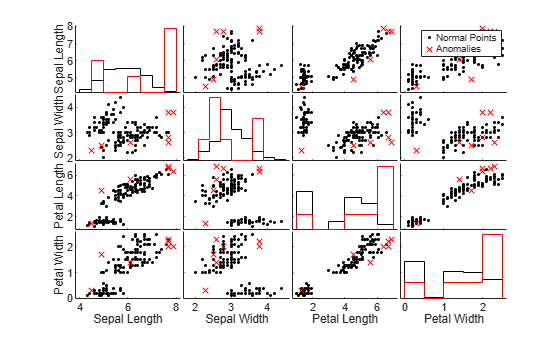## Model-Specific Anomaly Detection

Statistics and Machine Learning Toolbox™ provides model-specific anomaly detection features that you can apply after training a classification, regression, or clustering model. For example, you can detect anomalies by using these object functions:

• Proximity matrix — `outlierMeasure` for random forest (`CompactTreeBagger`)

• Mahalanobis distance — `mahal` for discriminant analysis classifier (`ClassificationDiscriminant`) and `mahal` for Gaussian mixture model (`gmdistribution`)

• Unconditional probability density — `logp` for discriminant analysis classifier (`ClassificationDiscriminant`), `logp` for naive Bayes classifier (`ClassificationNaiveBayes`), and `logp` for naive Bayes classifier for incremental learning (`incrementalClassificationNaiveBayes`)

For details, see the function reference pages.

### Detect Outliers After Training Random Forest

Train a random forest classifier by using the `TreeBagger` function, and detect outliers in the training data by using the object function `outlierMeasure`.

Train Random Forest Classifier

Load the `ionosphere` data set, which contains radar return qualities (`Y`) and predictor data (`X`) for 34 variables. Radar returns are either of good quality (`'g'`) or bad quality (`'b'`).

`load ionosphere`

Train a random forest classifier. Store the out-of-bag information for predictor importance estimation.

```rng("default") % For reproducibility Mdl_TB = TreeBagger(100,X,Y,Method="classification", ... OOBPredictorImportance="on");```

`Mdl_TB` is a `TreeBagger` model object for classification. `TreeBagger` stores predictor importance estimates in the property `OOBPermutedPredictorDeltaError`.

Detect Outliers Using Proximity

Detect outliers in the training data by using the `outlierMeasure` function. The function computes outlier measures based on the average squared proximity between one observation and the other observations in the trained random forest.

```CMdl_TB = compact(Mdl_TB); s_proximity = outlierMeasure(CMdl_TB,X,Labels=Y);```

A high value of the outlier measure indicates that the observation is an outlier. Find the threshold corresponding to the 95th percentile and identify outliers by using the `isoutlier` function.

`[TF,~,U] = isoutlier(s_proximity,Percentiles=[0 95]);`

Plot a histogram of the outlier measures. Create a vertical line at the outlier threshold.

```histogram(s_proximity) xline(U,"r-",join(["Threshold =" U])) title("Histogram of Outlier Measures")```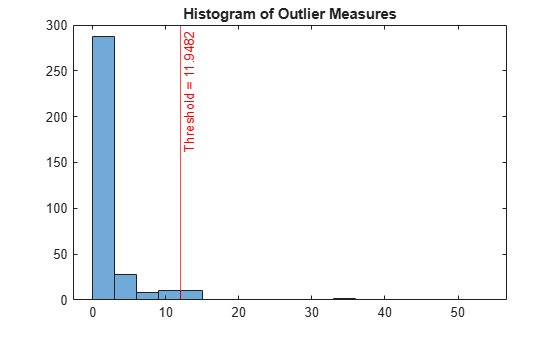Visualize observation values using the two most important features selected by the predictor importance estimates in the property `OOBPermutedPredictorDeltaError`.

```[~,idx] = sort(Mdl_TB.OOBPermutedPredictorDeltaError,'descend'); TF_c = categorical(TF,[0 1],["Normal Points" "Anomalies"]); gscatter(X(:,idx(1)),X(:,idx(2)),TF_c,"kr",".x",[],"on", ... Mdl_TB.PredictorNames(idx(1)),Mdl_TB.PredictorNames(idx(2)))```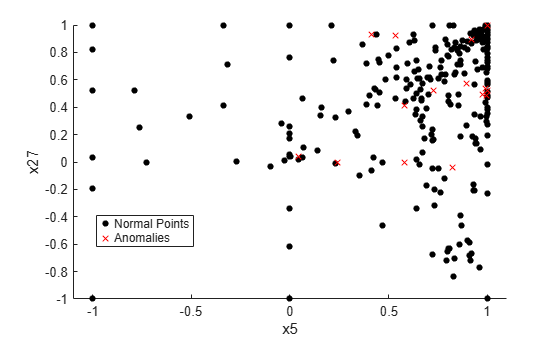Train the classifier again without outliers, and plot the histogram of the outlier measures.

```Mdl_TB = TreeBagger(100,X(~TF,:),Y(~TF),Method="classification"); s_proximity = outlierMeasure(CMdl_TB,X(~TF,:),Labels=Y(~TF)); histogram(s_proximity) title("Histogram of Outlier Measures After Removing Outliers")```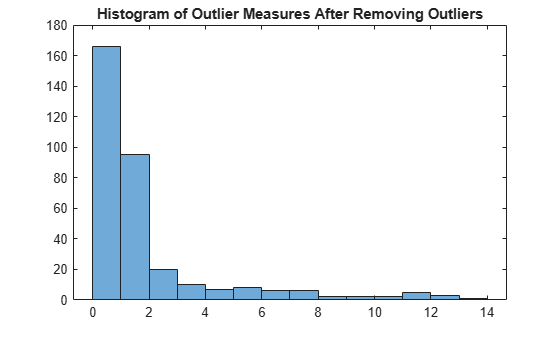### Detect Outliers After Training Discriminant Analysis Classifier

Train a discriminant analysis model by using the `fitcdiscr` function, and detect outliers in the training data by using the object functions `logp` and `mahal`.

Train Discriminant Analysis Model

Load Fisher's iris data set. The matrix `meas` contains flower measurements for 150 different flowers. The variable `species` lists the species for each flower.

`load fisheriris`

Train a discriminant analysis model using the entire data set.

```Mdl = fitcdiscr(meas,species,PredictorNames= ... ["Sepal Length" "Sepal Width" "Petal Length" "Petal Width"]);```

`Mdl` is a `ClassificationDiscriminant` model.

Detect Outliers Using Log Unconditional Probability Density

Compute the log unconditional probability densities of the training data.

`s_logp = logp(Mdl,meas);`

A low density value indicates that the corresponding observation is an outlier.

Determine the lower density threshold for outliers by using the `isoutlier` function.

`[~,L_logp] = isoutlier(s_logp);`

Identify outliers by using the threshold.

`TF_logp = s_logp < L_logp;`

Plot a histogram of the density values. Create a vertical line at the outlier threshold.

```figure histogram(s_logp) xline(L_logp,"r-",join(["Threshold =" L_logp])) title("Histogram of Log Unconditional Probability Densities ")```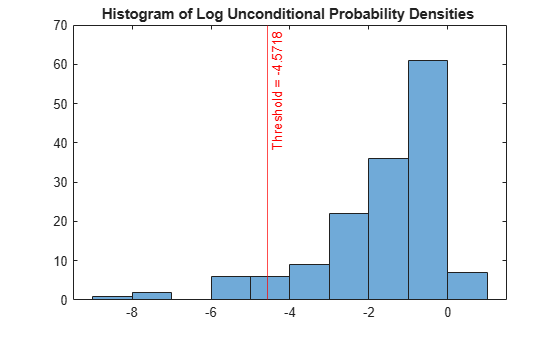To compare observation values between normal points and anomalies, create a matrix of grouped histograms and grouped scatter plots for each combination of variables by using the `gplotmatrix` function.

```TF_logp_c = categorical(TF_logp,[0 1],["Normal Points" "Anomalies"]); gplotmatrix(meas,[],TF_logp_c,"kr",".x",[],[],[],Mdl.PredictorNames)```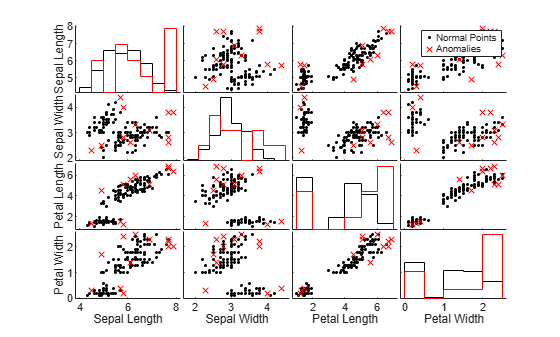Detect Outliers Using Mahalanobis Distance

Find the squared Mahalanobis distances from the training data to the class means of true labels.

`s_mahal = mahal(Mdl,meas,ClassLabels=species);`

A large distance value indicates that the corresponding observation is an outlier.

Determine the threshold corresponding to the 95th percentile and identify outliers by using the `isoutlier` function.

`[TF_mahal,~,U_mahal] = isoutlier(s_mahal,Percentiles=[0 95]);`

Plot a histogram of the distances. Create a vertical line at the outlier threshold.

```figure histogram(s_mahal) xline(U_mahal,"-r",join(["Threshold =" U_mahal])) title("Histogram of Mahalanobis Distances")```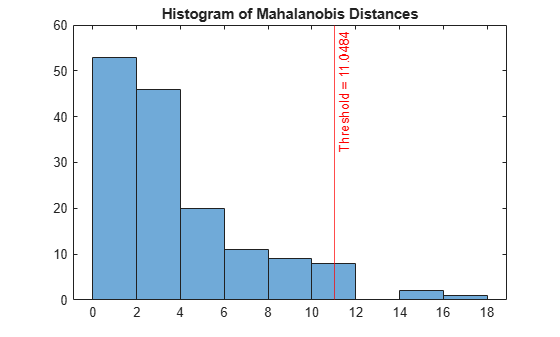Compare the observation values between normal points and anomalies by using the `gplotmatrix` function.

```TF_mahal_c = categorical(TF_mahal,[0 1],["Normal Points" "Anomalies"]); gplotmatrix(meas,[],TF_mahal_c,"kr",".x",[],[],[],Mdl.PredictorNames)```